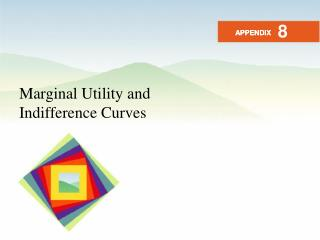# Marginal Utility and Indifference Curves - PowerPoint PPT PresentationDownload PresentationMarginal Utility and Indifference Curves

Marginal Utility and Indifference CurvesDownload Presentation## Marginal Utility and Indifference Curves

- - - - - - - - - - - - - - - - - - - - - - - - - - - E N D - - - - - - - - - - - - - - - - - - - - - - - - - - -
##### Presentation Transcript

1. 8 APPENDIX APPENDIX Marginal Utility and Indifference Curves

2. After studying this chapter you will be able to • Explain the connection between utility and indifference curves • Explain why maximizing utility is the same as choosing the best affordable point • Explain why utility exists

3. Two Ways of Describing Preferences • The marginal utility model describes preferences by using the concept of utility. • The indifference curve model describes preferences by using the concepts of preference and indifference. • Figure A8.1 on the next slide illustrates the connection between these two ways of describing preferences.

4. Two Ways of Describing Preferences • In part (a), you can see the levels of utility derived from each quantity of movies and soda. • Three combinations generate 331 units of utility and two combinations generate 313 units of utility. • Indifference curves pass through these points.

5. Maximizing Utility is Choosing the Best Affordable Point • Call the marginal utility of movies MUM . • Call the marginal utility of soda MUS . • Call the price of movies PM . • Call the price of soda PS . • The marginal utility per dollar from movies is MUM/PM . • The marginal utility per dollar from soda is MUS/PS. • Utility is maximized when • MUM/PM = MUS/PS.

6. Maximizing Utility is Choosing the Best Affordable Point • Call the marginal rate of substitution of movies for soda MRS. • The consumer is at the best affordable point on the budget line when • MRS = PM/PS.

7. Maximizing Utility is Choosing the Best Affordable Point • To see that maximizing utility is the same as choosing the best affordable point, begin with • MUM/PM = MUS/PS • and multiply both sides of this equation by PM and divide both sides by MUS to get • MUM/MUS = PM/PS.

8. Maximizing Utility is Choosing the Best Affordable Point • Because the best affordable point is when MRS = PM/PS, it must be the case that • MUM/MUS = MRS. • To see that this proposition is true, note first that • U = MUM QM + MUS QS . • But along an indifference curve, which is where we measure MRS, U = 0, so • 0 = MUM QM + MUS QS .

9. Maximizing Utility is Choosing the Best Affordable Point • Because • 0 = MUM QM + MUS QS • we know that • MUM QM = –MUS QS . • Now divide both sides of this equation by MUS and by QM to obtain • MUM / MUS = –QS /QM .

10. Maximizing Utility is Choosing the Best Affordable Point • But –QS /QM—rise over run—is the slope of the indifference curve and removing the minus sign, it is the marginal rate of substitution. • So • MRS = MUM / MUS = QS /QM . • The two models of consumer choice give the same answer. • One implies the other.

11. Utility Exists! • The indifference curve model is powerful because it enables us to derive the downward-sloping demand curve from the assumption of diminishing marginal rate of substitution. • The model is also powerful because it implies that utility exists. • By observing incomes and prices and the quantities bought at those prices, we can infer a person’s utility schedule and the marginal utilities at each quantity combination.

12. THE END